# Probability

### Term 2 starting in week 11 :: Estimated time: 2 weeks

• Know how to add, subtract and multiply fractions (review)
• Find probabilities using equally likely outcomes (review)
• Use the property that probabilities sum to 1 (review)
• Using experimental data to estimate probabilities
• Find probabilities from tables, Venn diagrams and frequency trees
• Construct and interpret sample spaces for more than one event (review)
• Calculate probability with independent events
• Use tree diagrams for independent events
• Use tree diagrams for dependent events

For higher-attaining pupils:

• Construct and interpret conditional probabilities (Tree diagrams)
• Construct and interpret conditional probabilities (Venn diagrams and two-way tables)

This page should remember your ticks from one visit to the next for a period of time. It does this by using Local Storage so the information is saved only on the computer you are working on right now.

## Lesson Starters

Here are some suggestions for whole-class, projectable resources which can be used at the beginnings of each lesson in this block.

### 1st Lesson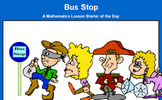#### Bus Stop

How many different ways can four people stand in line?

### 2nd Lesson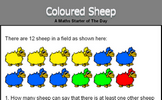#### Coloured Sheep

What is the probability of picking a red sheep from the sheep in the field?

### 3rd Lesson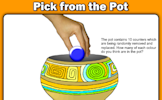#### Pick From The Pot

The pot contains 10 counters which are being randomly removed and replaced. How many of each colour do you think are in the pot?

### 4th Lesson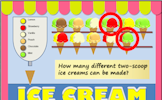#### Ice Cream

How many different ice cream cones can be made by choosing two scoops from six flavours?

### 5th Lesson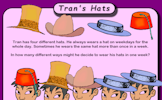#### Tran's Hats

In how many different ways might Tran decide to wear his hats in one week?

### 6th Lesson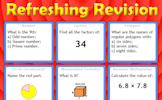#### Refreshing Revision

It is called Refreshing Revision because every time you refresh the page you get different revision questions.

Some of the Starters above are to reinforce concepts learnt, others are to introduce new ideas while others are on unrelated topics designed for retrieval practice or and opportunity to develop problem-solving skills.

White Rose ResourcesEnd of block assessments provide a quick progress check at the end of each block of learning to make sure students have understood the content covered. This Scheme of Learning was produced by White Rose Maths and is used here with permission granted on 30th June 2021.For All: